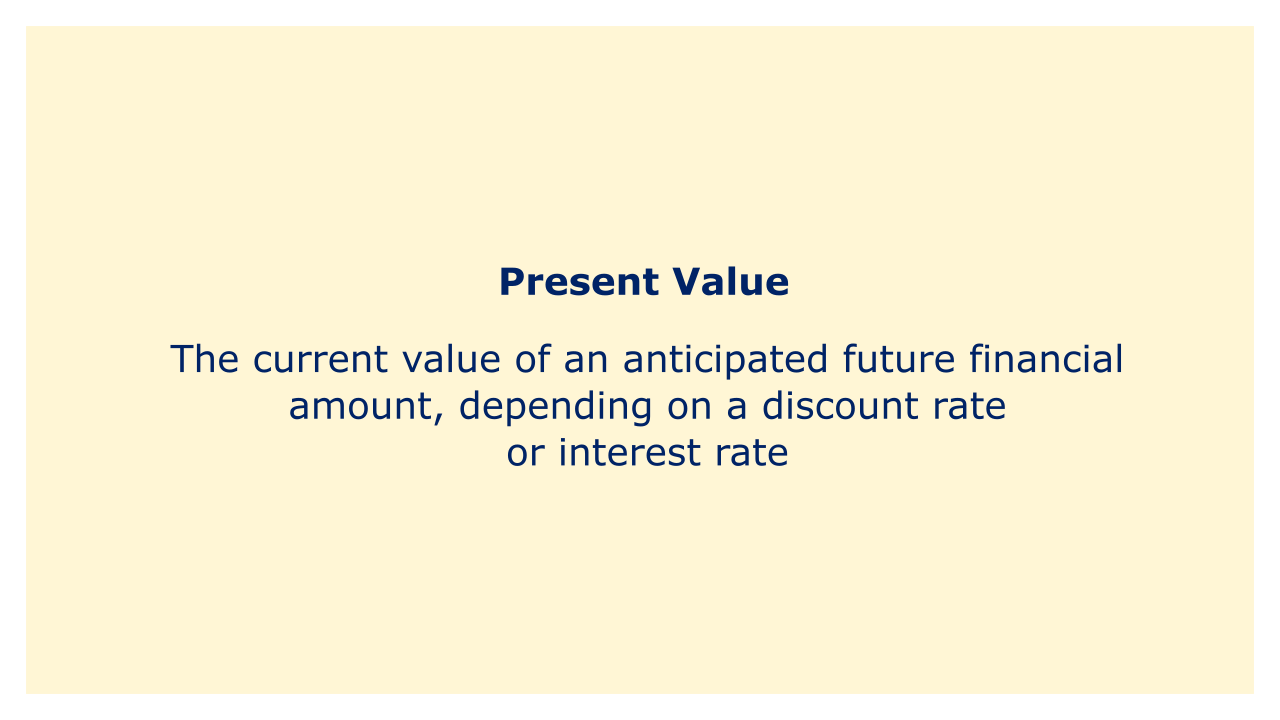# Present ValueImage: Moneybestpal.com

### The term "present value" in finance refers to the current value of an anticipated future financial amount, depending on a discount rate or interest rate. A dollar obtained in the future will be worth less than a dollar received today because the value of money fluctuates with time.

The present value calculation considers the time value of money and enables the comparison of cash flows that take place at various intervals. Investors can choose whether to pursue a given investment or project by discounting the future cash flows back to their present value. The valuation of financial assets like stocks, bonds, and options also employs the present value method.

The formula for calculating the present value is:

PV = FV / (1 + r)n

Where r is the discount rate, n is the number of time periods, PV is the present value, and FV is the future value. Given their desired rate of return or discount rate, investors can use this formula to calculate how much they ought to be willing to pay now for a future cash flow.
Tags